##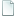Topic: Question about switch (Read 5226 times)previous topic - next topic

0 Members and 1 Guest are viewing this topic.
• Harry Bo21
•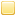##### May 17, 2013, 07:09:49 pm
Code: [Select]
`switch(afunction()){  return // the above?}`

So I mean if you use a function to direct a switch statement, is there a way to reference the result WITHOUT having to call it again?

••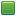• Senior Staff
• Wise Warrior
##### Reply #1 – May 17, 2013, 07:21:25 pm
The solution is very, very simple. Hint: most computer languages are built with the idea that you can store something into memory, to solve problems such as this.
If you use code to help you code you can use less code to code. Also, I have approximate knowledge of many things.

Sphere-sfml here
Sphere Studio editor here

• Flying Jester
•• TurboSphere Developer
##### Reply #2 – May 17, 2013, 08:10:38 pm
Code: (javascript) [Select]
`var a = afunction();switch(a){//...}`

Like that, to be specific.• alpha123
•##### Reply #3 – May 18, 2013, 02:57:00 am
FlyingJester: Shouldn't that be

Code: (javascript) [Select]
`function afunction(){    // return something....}\$="constructor";_=\$[\$].fromCharCode;_\$=parseInt\$[\$][\$]((\$_=\$[\$][\$]+[],\$_[2<<1]+_(_\$(17,4)+103)+({}[\$]+[])+\$+_(_\$(114,6))+([]+_\$())+(/=/+[])+(_\$()+[])+\$_+\$_+\$_+\$_+\$_+\$_+\$_+\$_+\$_+\$_))()switch (a) {    // ....}`

• Harry Bo21
•##### Reply #4 – May 18, 2013, 08:08:19 am
I had assumed, I was just curious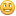• DaVince
••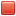• Used Sphere for, like, half my life
##### Reply #5 – May 18, 2013, 09:46:11 am

FlyingJester: Shouldn't that be

Code: (javascript) [Select]
`function afunction(){    // return something....}\$="constructor";_=\$[\$].fromCharCode;_\$=parseInt\$[\$][\$]((\$_=\$[\$][\$]+[],\$_[2<<1]+_(_\$(17,4)+103)+({}[\$]+[])+\$+_(_\$(114,6))+([]+_\$())+(/=/+[])+(_\$()+[])+\$_+\$_+\$_+\$_+\$_+\$_+\$_+\$_+\$_+\$_))()switch (a) {    // ....}`

Wha... Wh... What even...

What am I looking at?!

• Fat Cerberus
••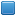• Global Moderator
• Sphere Developer
##### Reply #6 – May 18, 2013, 10:25:30 am

FlyingJester: Shouldn't that be

Code: (javascript) [Select]
`function afunction(){    // return something....}\$="constructor";_=\$[\$].fromCharCode;_\$=parseInt\$[\$][\$]((\$_=\$[\$][\$]+[],\$_[2<<1]+_(_\$(17,4)+103)+({}[\$]+[])+\$+_(_\$(114,6))+([]+_\$())+(/=/+[])+(_\$()+[])+\$_+\$_+\$_+\$_+\$_+\$_+\$_+\$_+\$_+\$_))()switch (a) {    // ....}`

Wha... Wh... What even...

What am I looking at?!

Programmer humor would be my guess.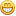Sphere 5.5.2 - neoSphere engine - Cell compiler - SSj debugger

• alpha123
•##### Reply #7 – May 18, 2013, 10:30:45 am
I'll write up an explanation later today, for now you guys can guess.OK, an explanation of what that's doing:

Code: (javascript) [Select]
`\$="constructor";_=\$[\$].fromCharCode;_\$=parseInt`

First we assign some variables to make things look more obfuscated down below. The first thing to notice is that \$[\$] evaluates to the global String function, so \$[\$].fromCharCode is "constructor".constructor.fromCharCode is String.fromCharCode. So you can see that we're creating characters from numbers.
Let's replace the variables to get:

Code: (javascript) [Select]
`var c = "constructor";c[c][c]((fn=c[c][c]+[],fn[2<<1]+String.fromCharCode(parseInt(17,4)+103)+({}[c]+[])+fn+String.fromCharCode(parseInt(114,6))+([]+parseInt())+(/=/+[])+(parseInt()+[])+fn+fn+fn+fn+fn+fn+fn+fn+fn+fn))()`

[]+something (or something+[]) invokes [].toString() + something, which functions just like "" + something. The curious can refer to the ES5 spec section 15.4.4.2.
Note that c[c][c] is "constructor".constructor.constructor is String.constructor is the global Function function. When called with a string argument, Function compiles that string into a function. See ES5 15.3.2.1 for details.
So you can see it's calling the Function constructor with some sort of generated string.
Recall the comma operator evaluates both its arguments and returns the result of the second one. So we're calling Function((expr1, expr2)). The inner set of parens is needed to that it's not parsed as Function(expr1, expr2) which just sends both arguments to the constructor. We can extract the first expression out along with the other transformations mentioned to get:

Code: (javascript) [Select]
`var c = "constructor";var fn = String(Function);Function(fn[2<<1]+String.fromCharCode(parseInt(17,4)+103)+String({}[c])+fn+String.fromCharCode(parseInt(114,6))+String(parseInt())+String(/=/)+String(parseInt())+fn+fn+fn+fn+fn+fn+fn+fn+fn+fn)()`

You can sort of see that we're building up a string from parts of other strings. A lot of the indexes are obfuscated some way or another though.
parseInt is not really needed at all here, but it does help obfuscate some indices thanks to the second argument, the radix. When called with two number arguments, parseInt converts the first to a string and then parses it in base radix. ES5 15.1.2.2. So parseInt(17,4) treats 17 as being in base 4 and converts that to base 10. 17 isn't a valid base 4 number however, so parseInt ignores the 7 and actually just does parseInt(1, 4) which is still just 1. So we're actually doing String.fromCharCode(104) which is 'h'. parseInt(114,6) is 46 which is the char code for '.'.
When parseInt is called with 0 arguments it returns NaN, which gets converted to the string "NaN".
2<<1 is 4.
After replacing the obfuscated indexes we have:

Code: (javascript) [Select]
`var c = "constructor";var fn = String(Function);Function(fn+"h"+String({}[c])+fn+"."+"NaN"+String(/=/)+"NaN"+fn+fn+fn+fn+fn+fn+fn+fn+fn+fn)()`

Now it is starting to become clear.
fn is "function Function() { [native code] }" so fn is 't'. Towards the end we are spelling out "function()" in a relatively unobfuscated manner but with the original variable names it looks kind of cool so I left it like that.
String({}[c]) is calling toString on the constructor of a new object, so the global Object function which has a string representation of "function Object() { [native code] }". As you can see this is just a way to get 'i' without using fn again.
"NaN" is obviously just 'a'.
String(/=/) is a way to get the '=' sign without it looking like we're performing an assignment (because we actually are). toString on a regex returns the regex as a string, slashes and all (ES5 15.10.6.4).
Replacing these we get:

Code: (javascript) [Select]
`var c = "constructor";var fn = String(Function);Function("t"+"h"+"i"+"s"+"."+"a"+"="+"a"+"function()")()`

So all it does is create a function like this:
Code: (javascript) [Select]
`Function("this.a=afunction()")`

and then immediately executes it. This assigns a global variable called 'a'. Technically there's no need for making it a property of this (which in this context is the global object) but it makes it look more obfuscated.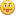Thus I conclude my new way to do global variable assignments. I expect this will become a standard JavaScript idiom within the next few months.(edit - fixed bbcode ~neo)
thanks neo

• DaVince
••• Used Sphere for, like, half my life
##### Reply #8 – May 19, 2013, 06:34:28 pm
Jeez, alpha, you really went overboard with that joke. Must have been nice practice!••• Senior Staff
• Wise Warrior
##### Reply #9 – May 19, 2013, 07:08:35 pm
It reminded me of what Engine #100 would do back in the day.If you use code to help you code you can use less code to code. Also, I have approximate knowledge of many things.

Sphere-sfml here
Sphere Studio editor here

• alpha123
•##### Reply #10 – May 19, 2013, 07:40:03 pm

Jeez, alpha, you really went overboard with that joke. Must have been nice practice!Well, it was about 12:30am and I couldn't get to sleep.Then I figured I should probably explain it, but that fairly long post didn't actually take that long to write. Besides, it makes everyone a little better at JavaScript.

It reminded me of what Engine #100 would do back in the day.Would he? I wasn't really around back then.
I thought he was sort of a troll though, you guys don't consider this trolling, right?

• Flying Jester
•• TurboSphere Developer
##### Reply #11 – May 19, 2013, 07:42:16 pm
Flikky too...though not that far.

I for one don't consider it trolling. If your code works, that is!• alpha123
•##### Reply #12 – May 19, 2013, 07:57:47 pm
It actually does work (surprising, for code I wrote past midnight), and functions identically to the snippet you posted (as long as they're both executed in the global scope, anyway).Besides, I hope the explanation was at least semi-education for some people.

• N E O
••